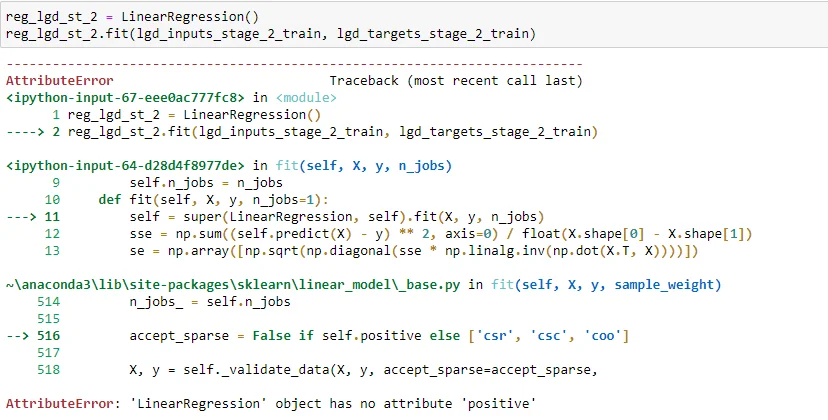24 Nov 2023

Posted on:

20 Jun 2021

3

# 'LinearRegression' object has no attribute 'positive'

Hi,

I have got this error while running the code in the lecture, any advise?Posted on:

30 Jun 2021

5

I have faced the same issue, but in my case it was when I created a derived class from LinearRegression that contained some extra functionality to calculate the p-value.
The missing part is initializing the base class during the initialization of the derived class, as I suspect the attribute "positive" has been added in a newer version.

`super().__init__(fit_intercept=fit_intercept, normalize=normalize, copy_X=copy_X, n_jobs=n_jobs)`

Here full code:

`class LinearRegression(linear_model.LinearRegression):`
`    """`
`    LinearRegression class after sklearn's, but calculate t-statistics`
`    and p-values for model coefficients (betas).`
`    Additional attributes available after .fit()`
`    are `t`and`p` which are of the shape (y.shape, X.shape)`
`    which is (n_features, n_coefs)`
`    This class sets the intercept to 0 by default, since usually we include it`
`    in X.`
`    """`

`    # nothing changes in __init__`
`    def __init__(self, fit_intercept=True, normalize=False, copy_X=True,`
`                 n_jobs=1):`
`        super().__init__(fit_intercept=fit_intercept, normalize=normalize, copy_X=copy_X, n_jobs=n_jobs)`
`        self.fit_intercept = fit_intercept`
`        self.normalize = normalize`
`        self.copy_X = copy_X`
`        self.n_jobs = n_jobs`

Posted on:

09 Sept 2021

0

`super().__init__(fit_intercept=fit_intercept, normalize=normalize, copy_X=copy_X, n_jobs=n_jobs, positive=False)`
`self.positive = positive`

'positive' = True, forces coefficient to be positive.

Posted on:

30 Mar 2022

0

I am getting the same error while running the code

``````AttributeError: 'LinearRegression' object has no attribute 'positive'
``````
Posted on:

30 Mar 2022

0

I also want to understand the concept behind the p-value class method.
What is the linear Algebra behind the logistic regression function?

Posted on:

24 Nov 2023

0

You need to update the code from Fernando a bit to make it work (at least on my end):

# nothing changes in __init__
def __init__(self, fit_intercept=True, normalize=False, copy_X=True,
n_jobs=1):
super().__init__(fit_intercept=fit_intercept, copy_X=copy_X, n_jobs=n_jobs)
self.fit_intercept = fit_intercept
self.normalize = normalize
self.copy_X = copy_X
self.n_jobs = n_jobs